# Go 线程调度器

## Go运行时为什么需要调度器？

POSIX的线程API是对现有Unix进程模型在逻辑上的一个强大大的扩展，使得线程得到了很多类似进程的控制能力。比如，线程有它自己的信号码，线程能够被赋予CPU affinity功能(就是指定线程只能在某个CPU上运行），线程能被添加到cgroups中，线程所占用的的资源也能够查询。这些额外特性大大增加了Go 程序在创建goroutine 时所需要的开销，是在线程多达100000的时候尤其明显。

## 来看看里面的各个角色（Our Cast of Characters）

Go试图通过M：N的调度器去获取这两种模型的全部优势。它在任意数目的OS线程上调用任意数目的goroutines。你可以快速进行上下文切换，并且还能利用你系统上所有的核心的优势。这个模型主要的缺点是它增加了调度器的复杂性。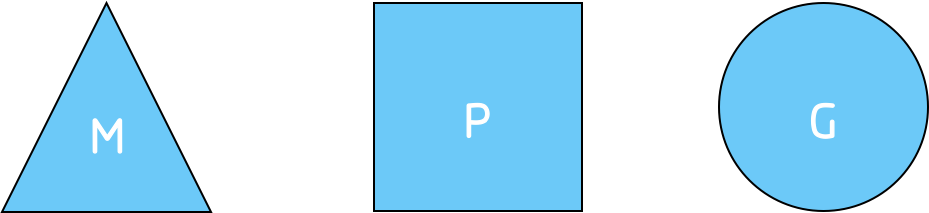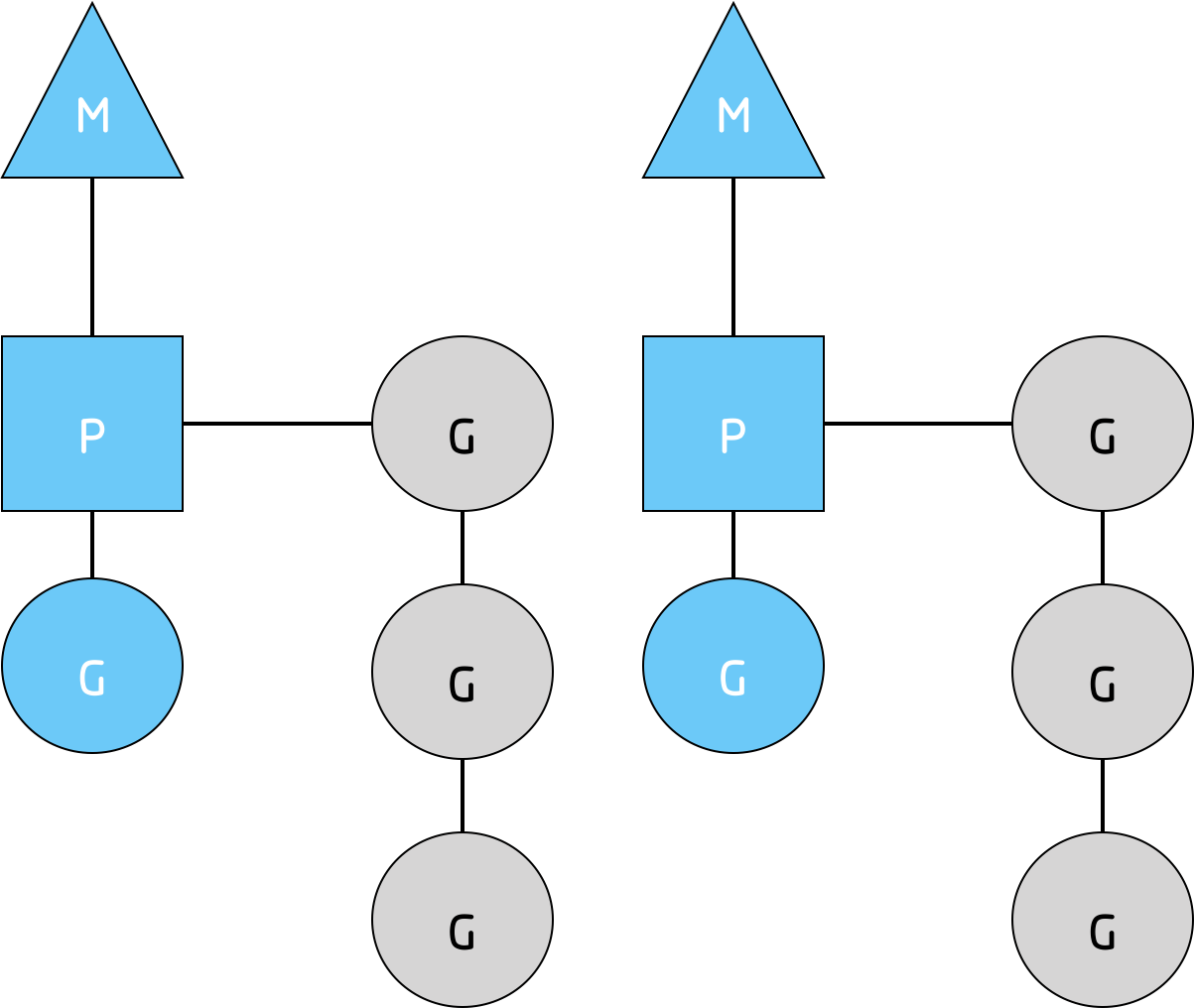## 系统调用细节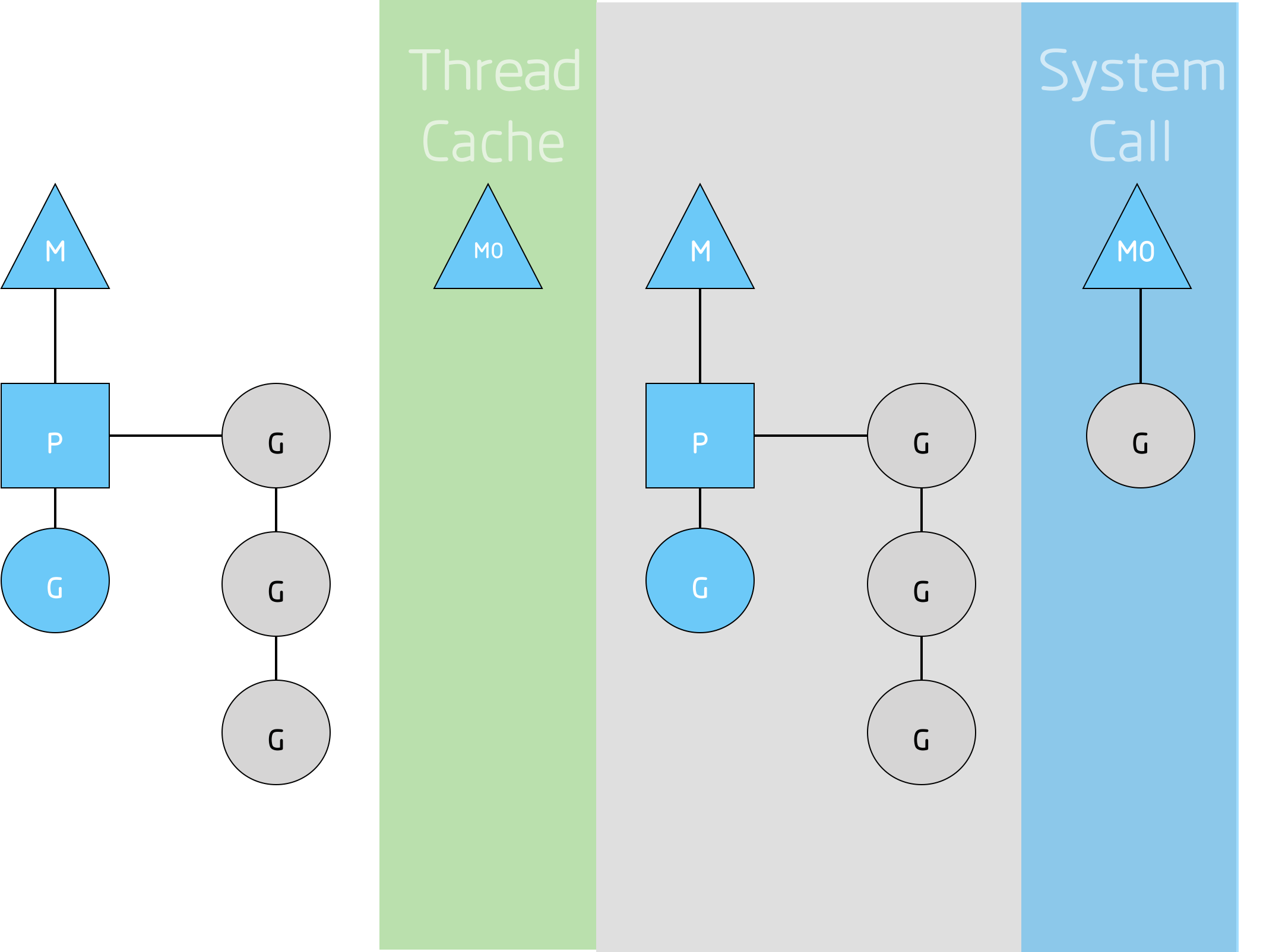Go程序要在多线程上运行就是因为要处理系统调用，哪怕`GOMAXPROCS`等于1。运行时使用调用系统调用的goroutines，而不是线程。

## 盗取工作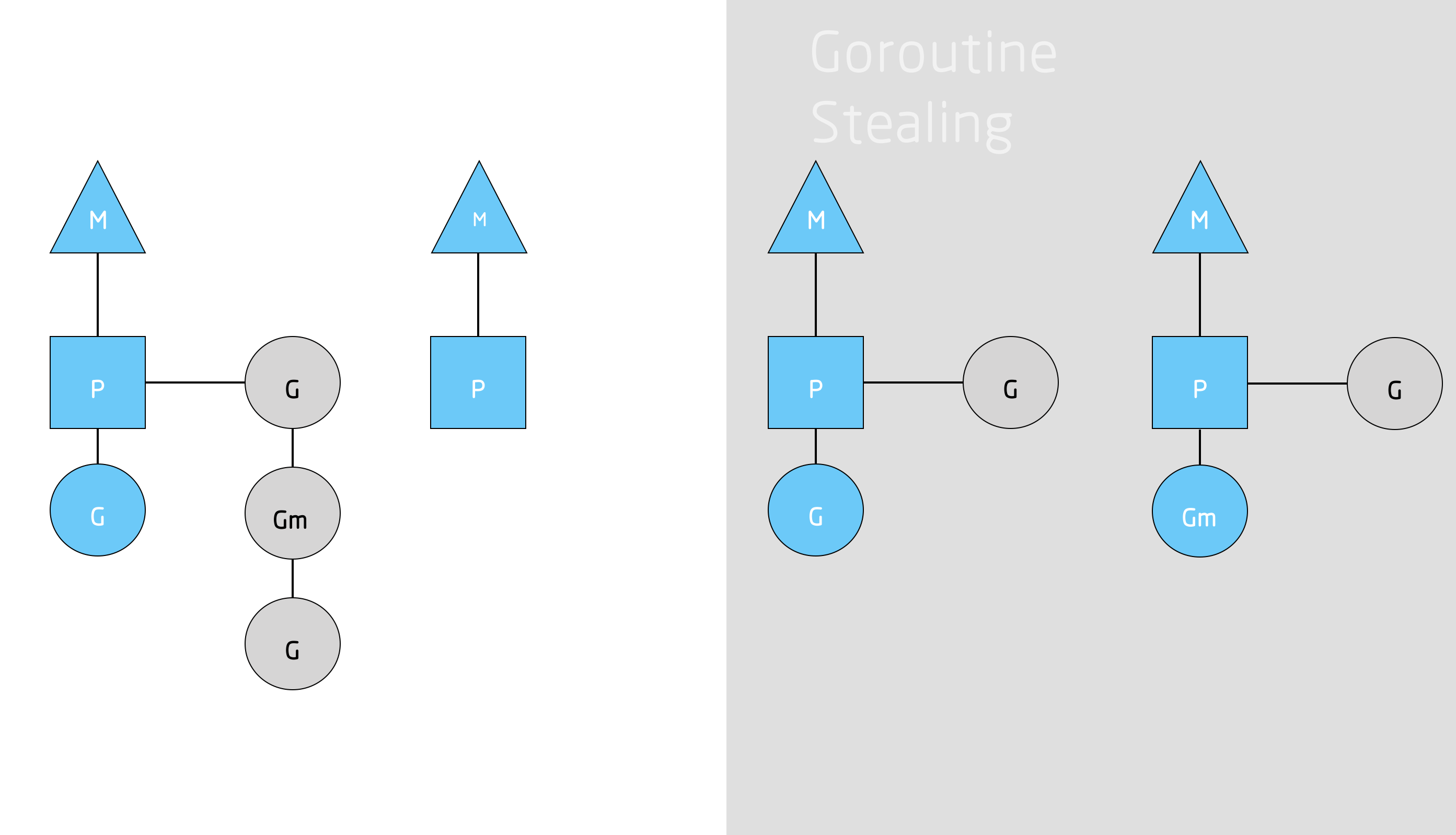## 展望

Releated articles:

# Lodash 源码分析（一）“Function” Methods

## 前言

Lodash一直是我很喜欢用的一个库，代码也十分简洁优美，一直想抽时间好好分析一下Lodash的源代码。最近抽出早上的一些时间来分析一下Lodash的一些我觉得比较好的源码。因为函数之间可能会有相互依赖，所以不会按照文档顺序进行分析，而是根据依赖关系和简易程度由浅入深地进行分析。因为个人能力有限，如果理解有偏差，还请直接指出，以便我及时修改。

##`_.after`

`_.after`函数几乎是Lodash中最容易理解的一个函数了，它一共有两个参数，第一个参数是调用次数`n`,第二个参数是`n`次调用之后执行的函数`func`

``````function after(n, func) {
if (typeof func != 'function') {
throw new TypeError(FUNC_ERROR_TEXT);
}
n = toInteger(n);
return function() {
if (--n < 1) {
return func.apply(this, arguments);
}
};
}
``````

``````func.apply(this,arguments);
``````

``````return function() {
if (--n < 1) {
return func.apply(this, arguments);
}
};
``````

`_.after`函数调用后返回了另一个函数，所以对于`_.after`函数的返回值，我们是需要再次调用的。所以最好的场景可能是在延迟加载等场景中。当然为了简单起见我给出一个很简单的例子：

``````const _ = require("lodash");

function foo(func ){
console.log("invoked foo.");
func();
}

var done = _.after(2,function bar(){
console.log("invoke bar");
});

for( var i = 0; i <  4; i++ ){
foo(done);
}
``````

``````invoked foo
invoked foo
invoke bar
invoked foo
invoke bar
invoked foo
invoke bar
``````

``````const _ = require("lodash");

function foo(func ){
this.name = "foo";
console.log("invoked foo: " + this.name );
func();
}

var done = _.after(2,function bar(){
console.log("invoke bar: " + this.name);
});

for( var i = 0; i <  4; i++ ){
foo(done);
}
``````

``````invoked foo: foo
invoked foo: foo
invoke bar: foo
invoked foo: foo
invoke bar: foo
invoked foo: foo
invoke bar: foo
``````

## `_.map`

`_.map`函数我们几乎随处可见，这个函数应用也相当广泛。

``````function map(collection, iteratee) {
var func = isArray(collection) ? arrayMap : baseMap;
return func(collection, getIteratee(iteratee, 3));
}
``````

``````_.map([1,2,3],func);
``````

``````function arrayMap(array, iteratee) {
var index = -1,
length = array == null ? 0 : array.length,
result = Array(length);

while (++index < length) {
result[index] = iteratee(array[index], index, array);
}
return result;
}
``````

``````function getIteratee() {
var result = lodash.iteratee || iteratee;
result = result === iteratee ? baseIteratee : result;
return arguments.length ? result(arguments, arguments) : result;
}
``````

``````   function baseIteratee(value) {
// Don't store the `typeof` result in a variable to avoid a JIT bug in Safari 9.
// See https://bugs.webkit.org/show_bug.cgi?id=156034 for more details.
if (typeof value == 'function') {
return value;
}
if (value == null) {
return identity;
}
if (typeof value == 'object') {
return isArray(value)
? baseMatchesProperty(value, value)
: baseMatches(value);
}
return property(value);
}
``````

``````function map(collection,iteratee){
return arrayMap(collection,getIteratee(iteratee,3));
}

function arrayMap(array, iteratee) {
var index = -1,
length = array == null ? 0 : array.length,
result = Array(length);

while (++index < length) {
result[index] = iteratee(array[index], index, array);
}
return result;
}

function getIteratee() {
var result =  baseIteratee;
return arguments.length ? result(arguments, arguments) : result;
}

function baseIteratee(value) {
if (typeof value == 'function') {
return value;
}
}
``````

``````function func(){
console.log(“arguments ” + arguments);
console.log(“arguments ” + arguments);
console.log(“arguments ” + arguments);
console.log("-----")
}
``````

``````arguments 6
arguments 0
arguments 6,8,10
-----
arguments 8
arguments 1
arguments 6,8,10
-----
arguments 10
arguments 2
arguments 6,8,10
-----
[ undefined, undefined, undefined ]
``````

## `_.ary`

``````const _ = require("lodash");
_.map(['6','8','10'],parseInt);
``````

``````[ 6, NaN, 2 ]
``````

``````/**
* @param string 必需。要被解析的字符串。
* 可选。表示要解析的数字的基数。该值介于 2 ~ 36 之间。
* 如果省略该参数或其值为 0，则数字将以 10 为基础来解析。如果它以 “0x” 或 “0X” 开头，将以 16 为基数。
* 如果该参数小于 2 或者大于 36，则 parseInt() 将返回 NaN
*/
/**

*/
``````

``````const _ = require("lodash");
_.map(['6','8','10'],_.ary(parseInt),1);
``````

`````` function ary(func, n, guard) {
n = guard ? undefined : n;
n = (func && n == null) ? func.length : n;
return createWrap(func, WRAP_ARY_FLAG, undefined, undefined, undefined, undefined, n);
}
``````

``````/**
* Creates a function that either curries or invokes `func` with optional
* `this` binding and partially applied arguments.
*
* @private
* @param {Function|string} func The function or method name to wrap.
*    1 - `_.bind` 1                      0b0000000000000001
*    2 - `_.bindKey`                     0b0000000000000010
*    4 - `_.curry` or `_.curryRight`...  0b0000000000000100
*    8 - `_.curry`                       0b0000000000001000
*   16 - `_.curryRight`                  0b0000000000010000
*   32 - `_.partial`                     0b0000000000100000
*   64 - `_.partialRight`                0b0000000001000000
*  128 - `_.rearg`                       0b0000000010000000
*  256 - `_.ary`                         0b0000000100000000
*  512 - `_.flip`                        0b0000001000000000
* @param {*} [thisArg] The `this` binding of `func`.
* @param {Array} [partials] The arguments to be partially applied.
* @param {Array} [holders] The `partials` placeholder indexes.
* @param {Array} [argPos] The argument positions of the new function.
* @param {number} [ary] The arity cap of `func`.
* @param {number} [arity] The arity of `func`.
* @returns {Function} Returns the new wrapped function.
*/
function createWrap(func, bitmask, thisArg, partials, holders, argPos, ary, arity) {
var isBindKey = bitmask & WRAP_BIND_KEY_FLAG;
if (!isBindKey && typeof func != 'function') {
throw new TypeError(FUNC_ERROR_TEXT);
}
var length = partials ? partials.length : 0;
if (!length) {
partials = holders = undefined;
}
ary = ary === undefined ? ary : nativeMax(toInteger(ary), 0);
arity = arity === undefined ? arity : toInteger(arity);
length -= holders ? holders.length : 0;

var partialsRight = partials,
holdersRight = holders;

partials = holders = undefined;
}
var data = isBindKey ? undefined : getData(func);

var newData = [
func, bitmask, thisArg, partials, holders, partialsRight, holdersRight,
argPos, ary, arity
];

if (data) {
mergeData(newData, data);
}
func = newData;
thisArg = newData;
partials = newData;
holders = newData;
arity = newData = newData === undefined
? (isBindKey ? 0 : func.length)
: nativeMax(newData - length, 0);

if (!arity && bitmask & (WRAP_CURRY_FLAG | WRAP_CURRY_RIGHT_FLAG)) {
}
var result = createBind(func, bitmask, thisArg);
} else if ((bitmask == WRAP_PARTIAL_FLAG || bitmask == (WRAP_BIND_FLAG | WRAP_PARTIAL_FLAG)) && !holders.length) {
result = createPartial(func, bitmask, thisArg, partials);
} else {
result = createHybrid.apply(undefined, newData);
}
var setter = data ? baseSetData : setData;
}
``````

``````function createWrap(func, bitmask, thisArg, partials, holders, argPos, ary, arity) {
//      0000000100000000 & 0000000000000010
// var isBindKey = bitmask & WRAP_BIND_KEY_FLAG;
var isBindKey = 0;
var length =  0;
// if (!length) {
//              0000000000100000 | 0000000001000000
//            ~(0000000001100000)
//              1111111110011111
//             &0000000100000000
//              0000000100000000 = WRAP_ARY_FLAG
// bitmask &= ~(WRAP_PARTIAL_FLAG | WRAP_PARTIAL_RIGHT_FLAG);
//  partials = holders = undefined;
// }
partials = holders = undefined;
ary = undefined;
arity = arity === undefined ? arity : toInteger(arity);
// because holders == undefined
//length -= 0;
// because isBindKey  == 0
// var data = isBindKey ? undefined : getData(func);
var data = getData(func);
var newData = [
func, bitmask, thisArg, partials, holders, partialsRight, holdersRight,
argPos, ary, arity
];
if (data) {
mergeData(newData, data);
}
func = newData;
thisArg = newData;
partials = newData;
holders = newData;
arity = newData = newData === undefined
? func.length : newData;
result = createHybrid.apply(undefined, newData);
var setter = data ? baseSetData : setData;
}
``````

``````   function createHybrid(func, bitmask, thisArg, partials, holders, partialsRight, holdersRight, argPos, ary, arity) {
var isAry = bitmask & WRAP_ARY_FLAG,
isCurried = bitmask & (WRAP_CURRY_FLAG | WRAP_CURRY_RIGHT_FLAG),
Ctor = isBindKey ? undefined : createCtor(func);

function wrapper() {
var length = arguments.length,
args = Array(length),
index = length;

while (index--) {
args[index] = arguments[index];
}
if (isCurried) {
var placeholder = getHolder(wrapper),
holdersCount = countHolders(args, placeholder);
}
if (partials) {
args = composeArgs(args, partials, holders, isCurried);
}
if (partialsRight) {
args = composeArgsRight(args, partialsRight, holdersRight, isCurried);
}
length -= holdersCount;
if (isCurried && length < arity) {
var newHolders = replaceHolders(args, placeholder);
return createRecurry(
args, newHolders, argPos, ary, arity - length
);
}
var thisBinding = isBind ? thisArg : this,
fn = isBindKey ? thisBinding[func] : func;

length = args.length;
if (argPos) {
args = reorder(args, argPos);
} else if (isFlip && length > 1) {
args.reverse();
}
if (isAry && ary < length) {
args.length = ary;
}
if (this && this !== root && this instanceof wrapper) {
fn = Ctor || createCtor(fn);
}
return fn.apply(thisBinding, args);
}
return wrapper;
}
``````

``````   function createHybrid(func, bitmask, thisArg, partials, holders, partialsRight, holdersRight, argPos, ary, arity) {
var isAry = 1;
function wrapper() {
var length = arguments.length,
args = Array(length),
index = length;
while (index--) {
args[index] = arguments[index];
}
var thisBinding = this, fn = func;
length = args.length;
if (isAry && ary < length) {
args.length = ary;
}
return fn.apply(thisBinding, args);
}
return wrapper;
}
``````

``````function ary(func,n){
return function(){
var length = arguments.length,
args = Array(length),
index = length;
while(index--){
args[index] = arguments[index];
}
args.length = n;
return func.apply(this,args);
}
}
``````

``````console.log(_.map(['6','8','10'],ary(parseInt,1)));
``````

``````[ 6, 8, 10 ]
``````

# Go实时GC——三色算法理论与实践

Go语言能够支持实时的，高并发的消息系统，在高达百万级别的消息系统中能够将延迟降低到100ms以下，着一切很大一部分需要归功于Go的高效的垃圾回收系统。

## 并行垃圾回收是如何工作的?

Go的GC是如何实现并行的呢？其中的关键在于`tricolor mark-and-sweep algorithm` 三色标记清除算法。该算法能够让系统的gc暂停时间成为能够预测的问题。调度器能够在很短的时间内实现GC调度，并且对源程序的影响极小。下面我们看看三色标记清除算法是如何工作的：

``````var A LinkedListNode;
// ...
// ...
B.next = *(B.next).next;
B.next = nil;
``````

### 第一步

``````var A LinkedListNode;

// ...

``````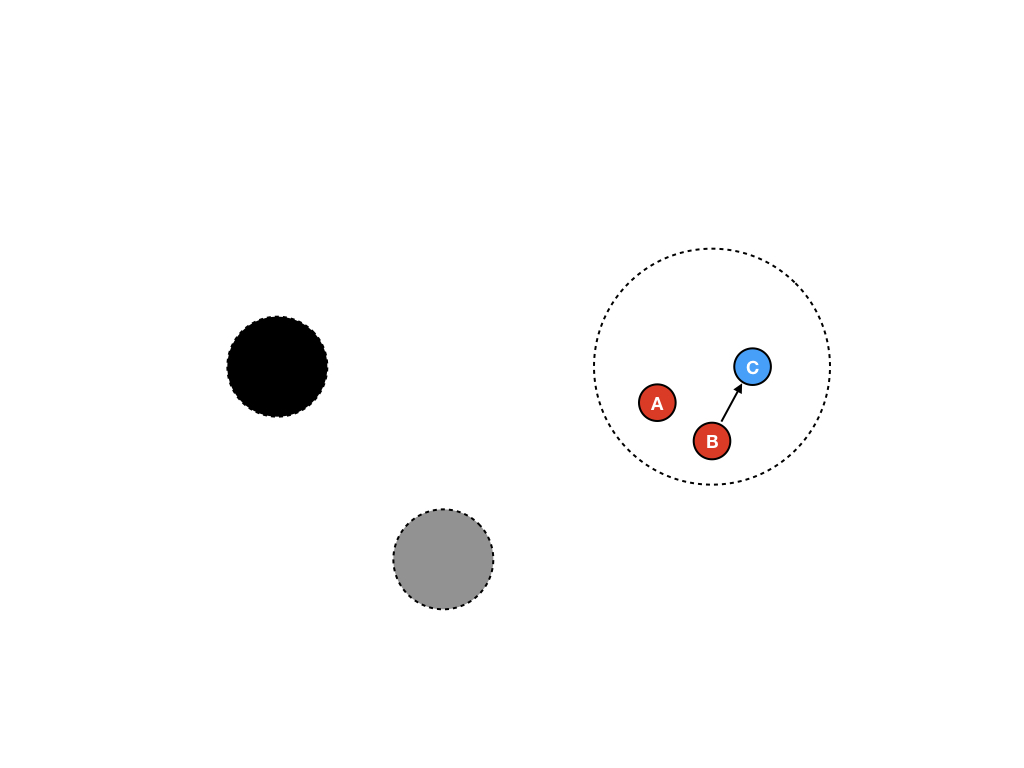### 第二步

``````var A LinkedListNode;

// ...

// ...
``````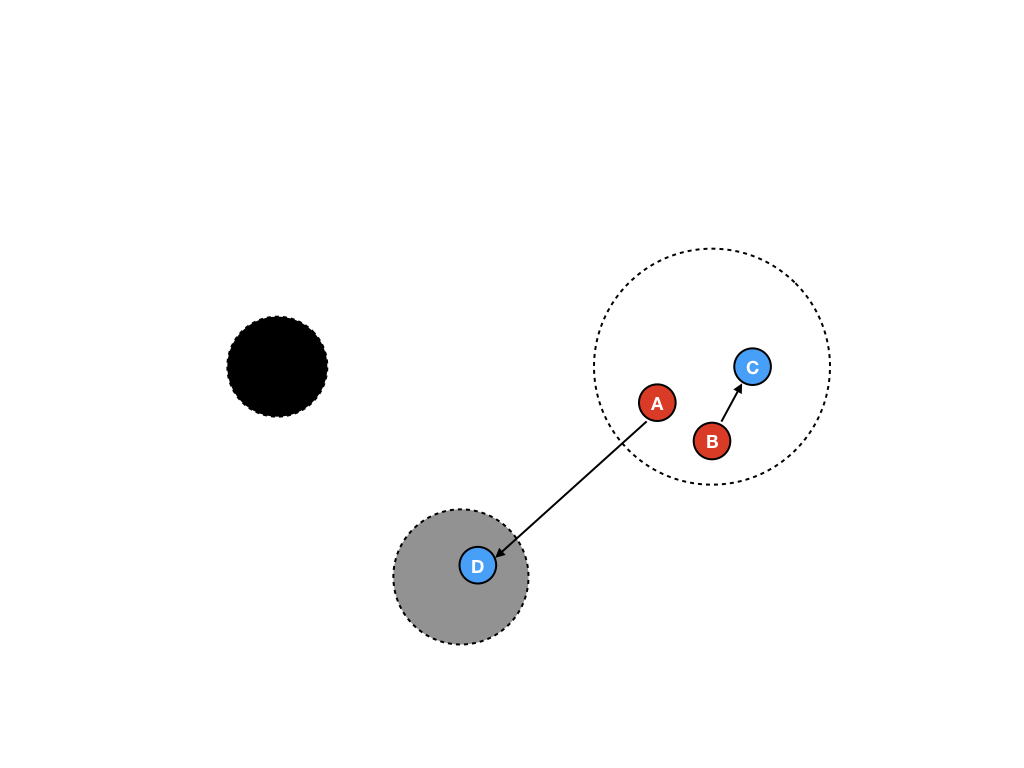### 第三步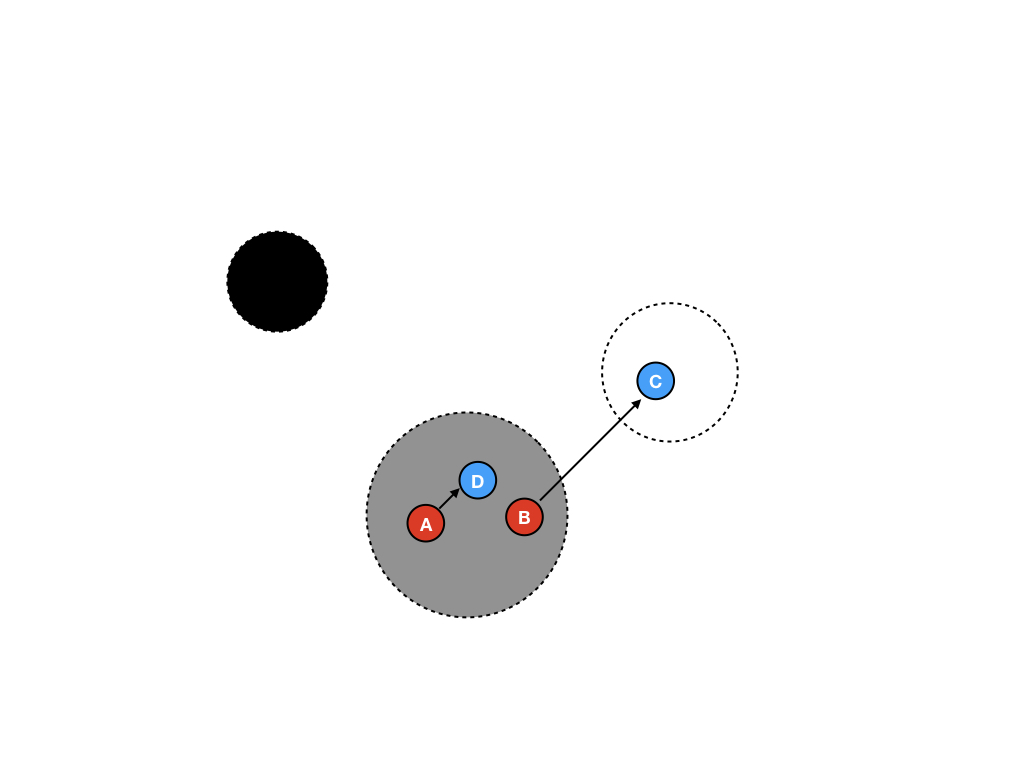### 第四步 扫描内存对象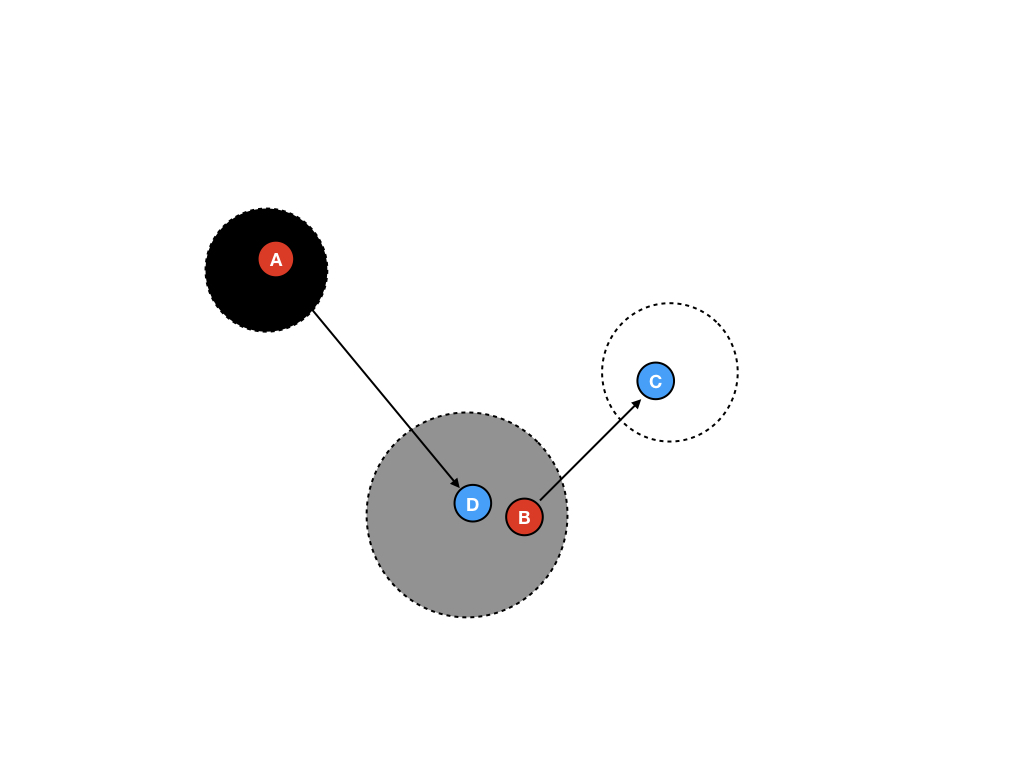### 第五步

``````var A LinkedListNode;

// ...

// ...
//新赋值 C.next = &E
``````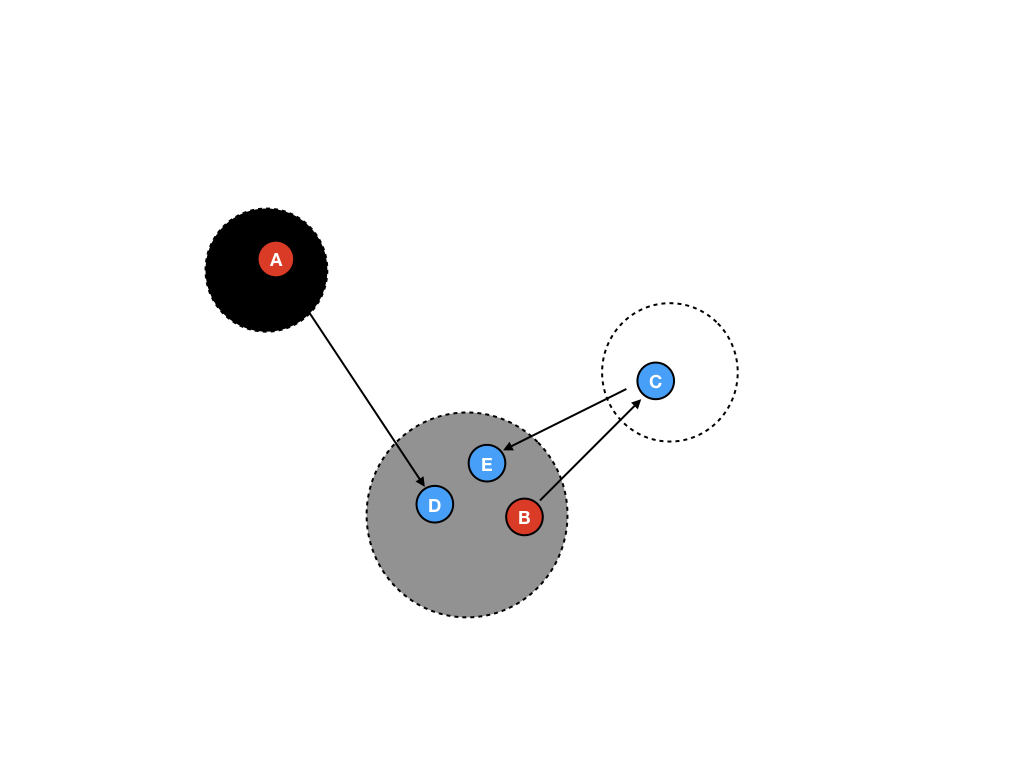### 第六步 指针重新赋值

``````var A LinkedListNode;
// ...
// ...
// 指针重新赋值:
B.next = *(B.next).next;
``````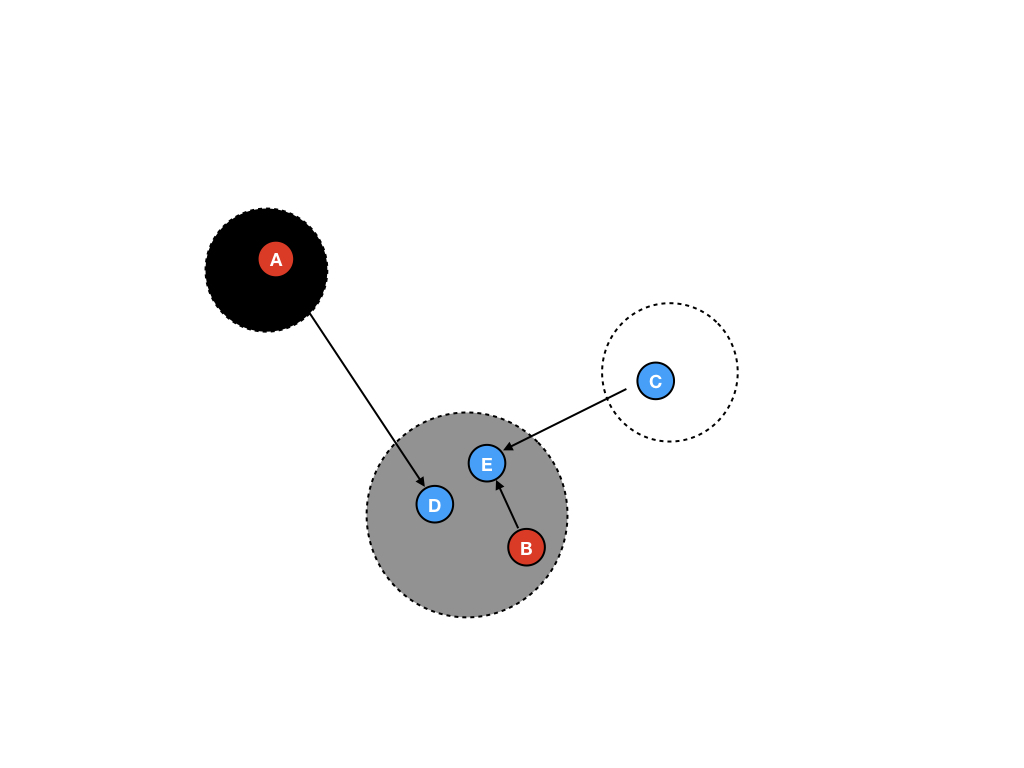### 第七步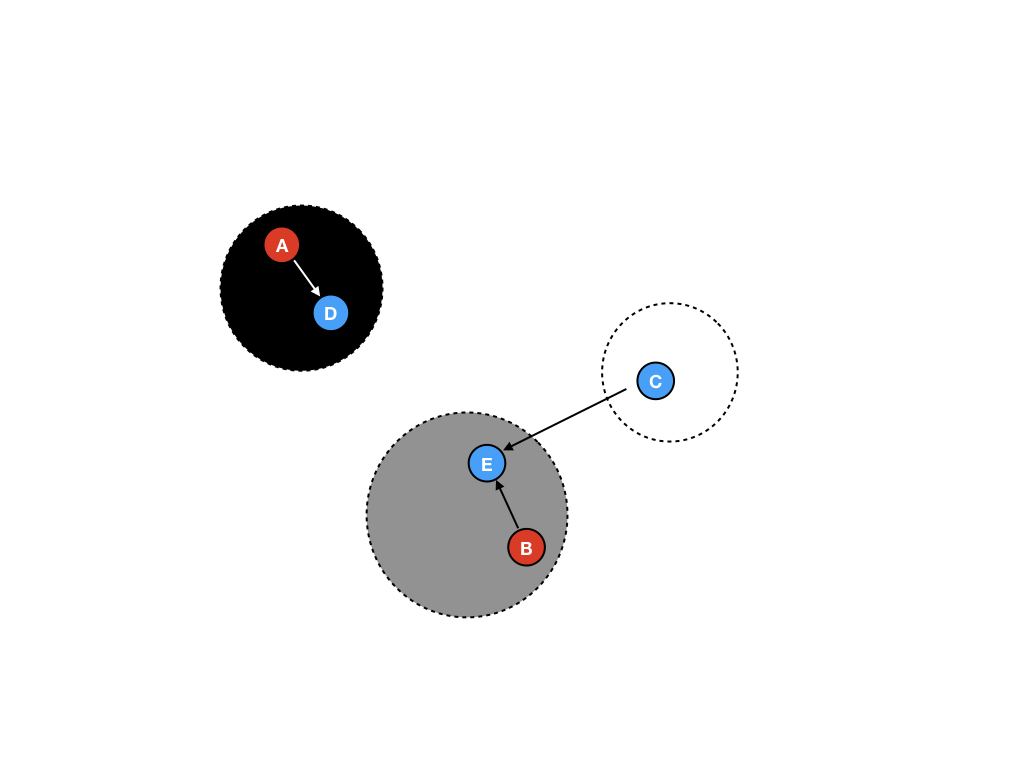### 第八步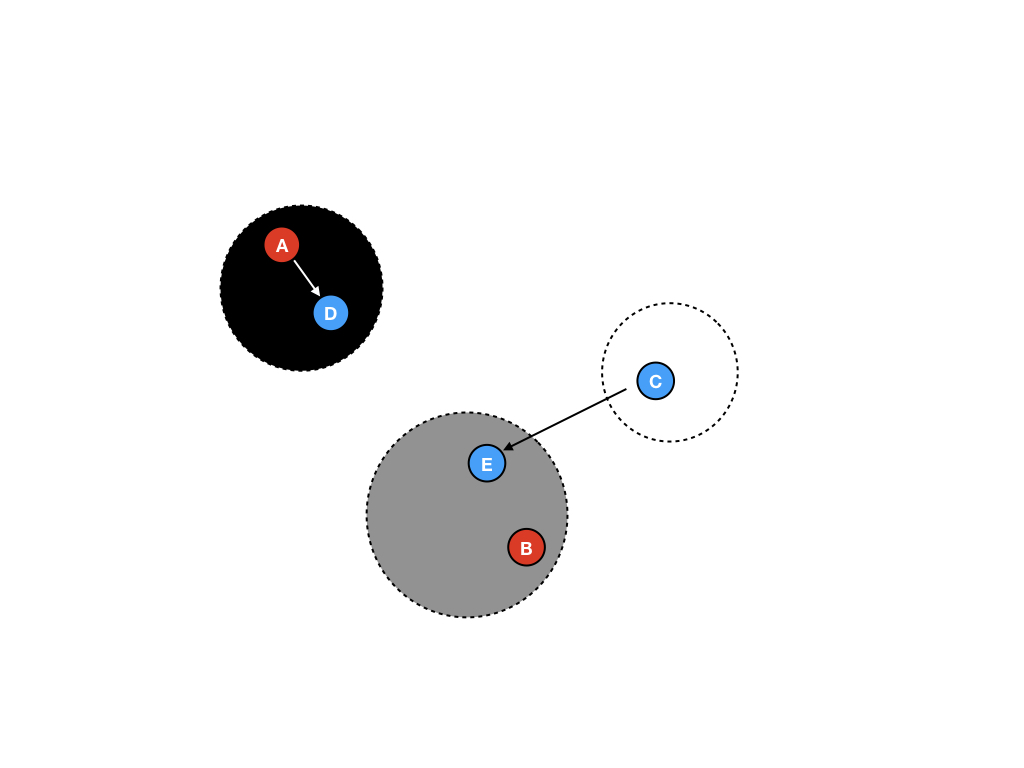### 第九步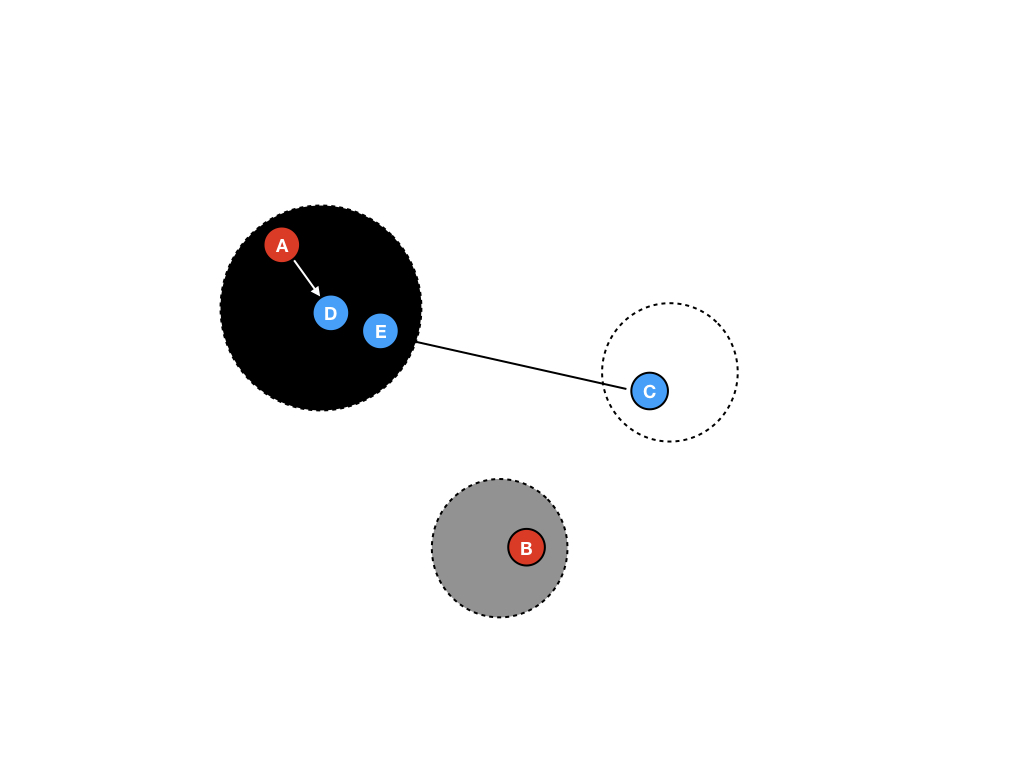### 第十步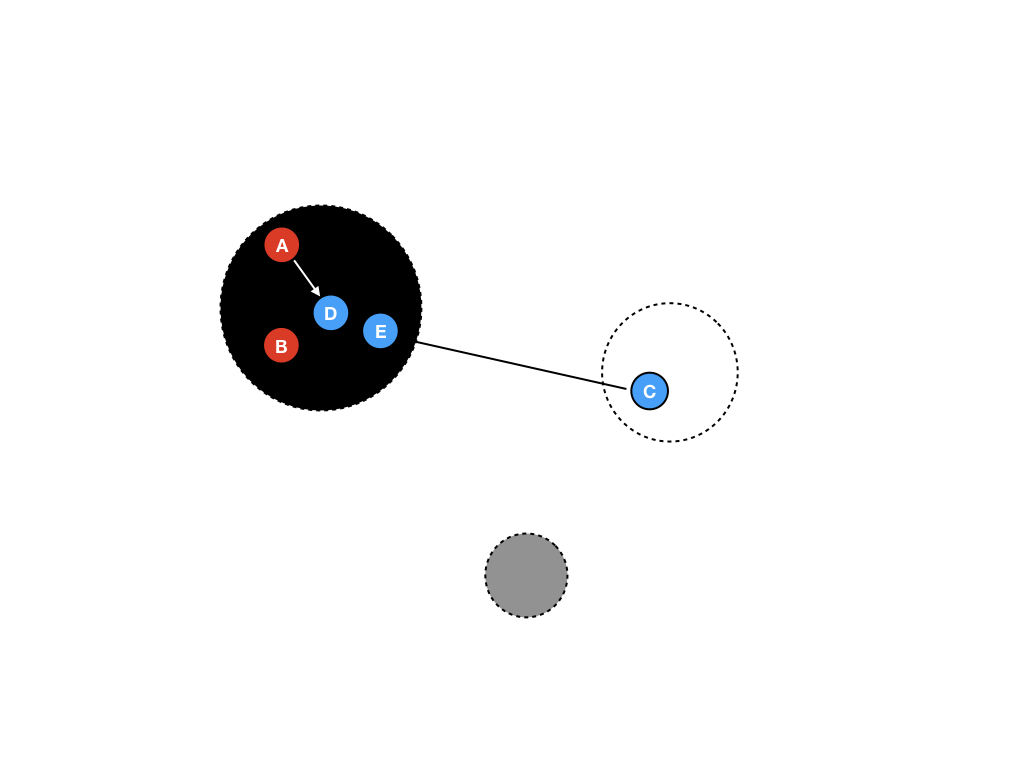### 第十一步 回收白色区域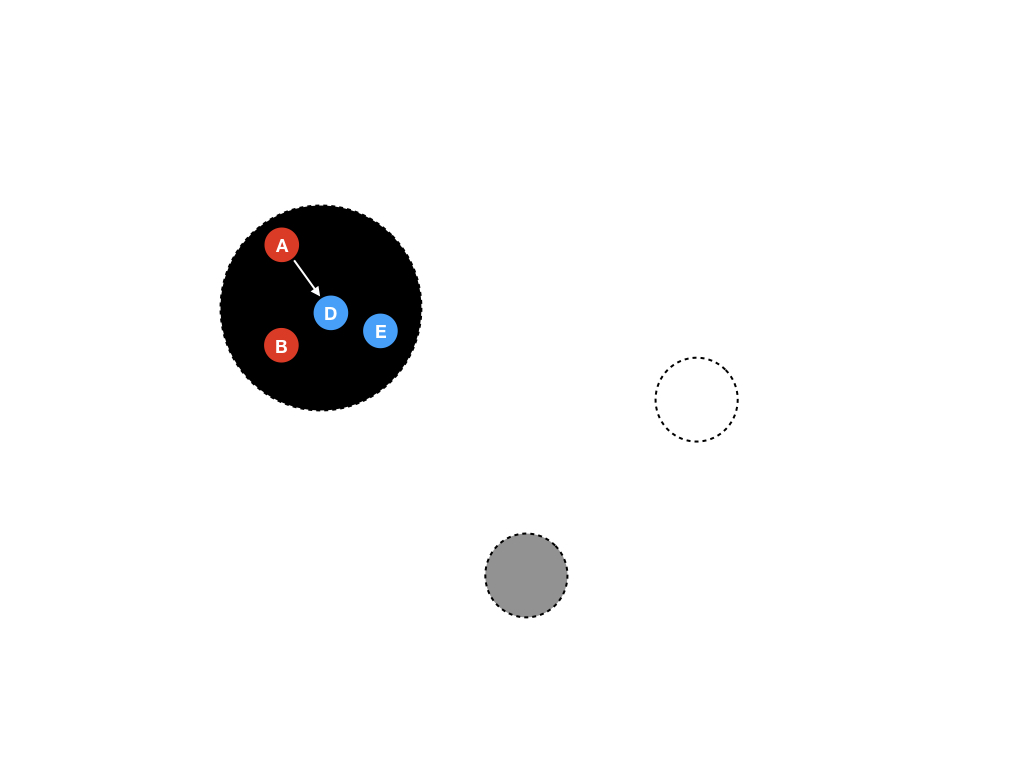### 第十二步 区域变色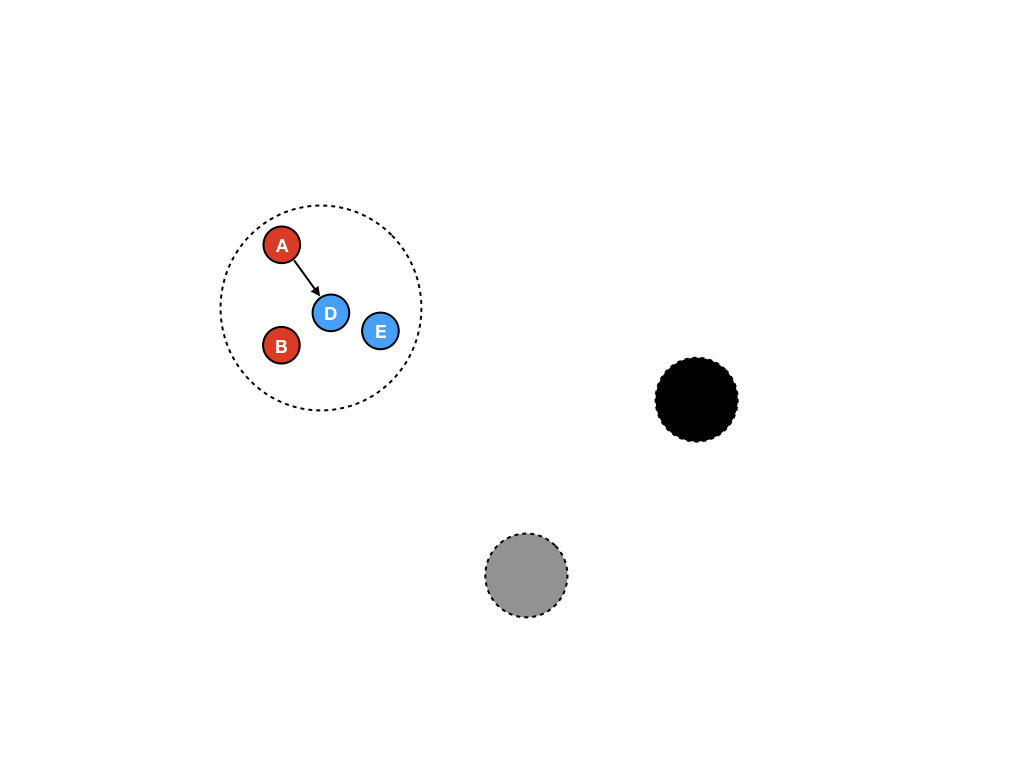## 实际表现

``````package main

import (
"fmt"
"time"
)

const (
windowSize = 200000
msgCount   = 1000000
)

type (
message []byte
buffer  [windowSize]message
)

var worst time.Duration

func mkMessage(n int) message {
m := make(message, 1024)
for i := range m {
m[i] = byte(n)
}
return m
}

func pushMsg(b *buffer, highID int) {
start := time.Now()
m := mkMessage(highID)
(*b)[highID%windowSize] = m
elapsed := time.Since(start)
if elapsed > worst {
worst = elapsed
}
}

func main() {
var b buffer
for i := 0; i < msgCount; i++ {
pushMsg(&b, i)
}
fmt.Println("Worst push time: ", worst)
}
``````

Benchmark Longest pause (ms)
OCaml 4.03.0 (map based) (manual timing) 2.21
Haskell/GHC 8.0.1 (map based) (rts timing) 67.00
Haskell/GHC 8.0.1 (array based) (rts timing) 58.60
Racket 6.6 experimental incremental GC (map based) (tuned) (rts timing) 144.21
Racket 6.6 experimental incremental GC (map based) (untuned) (rts timing) 124.14
Racket 6.6 (map based) (tuned) (rts timing) 113.52
Racket 6.6 (map based) (untuned) (rts timing) 136.76
Go 1.7.3 (array based) (manual timing) 7.01
Go 1.7.3 (map based) (manual timing) 37.67
Go HEAD (map based) (manual timing) 7.81
Java 1.8.0_102 (map based) (rts timing) 161.55
Java 1.8.0_102 G1 GC (map based) (rts timing) 153.89

### 一些注意事项

1. 进行基准测试往往需要多加小心，因为不同的运行时针对不同的测试用例都有不同程度的优化，所以表现往往也有差异。而我们需要针对自己的需求来编写测试用例，对于基准测试应该能够满足我们自己的产品需求。在上面的例子中可以看到，Go已经完全能够满足我们的产品需求。
2. Map Vs. Array： 最初我们的基准测试是在map中进行插入和删除操作的，但是Go在对大型的map进行GC的时候存在Bug。因此在设计Go的基准测试的时候用可修改的Array作为Map的替代。Go map的Bug已经在1.8版本中得到了修复，但是并不是所有的基准测试都得到了修正，这也是我们需要正视的一些问题。但是不管怎么说，没有理由说GC时间将会因为使用map导致大幅度增长（除去bug和糟糕的实现之外）。
3. manual timing Vs. rst timing :作为另一个注意事项，有些基准测试则在不同的计时系统下将会有所差异，因为有些语言不支持运行时时间统计，例如Go，而有些语言则支持。因此，我们应该在测试时候都把计时方式设置为manual timing。
4. 最后一个需要注意的事项是测试用例的实现将会极大地影响基准测试的结果，如果map的插入删除实现方式比较糟糕，则将会对测试结果造成不利影响，这也是用array的另一个原因。

## 为什么Go的结果不能再好点？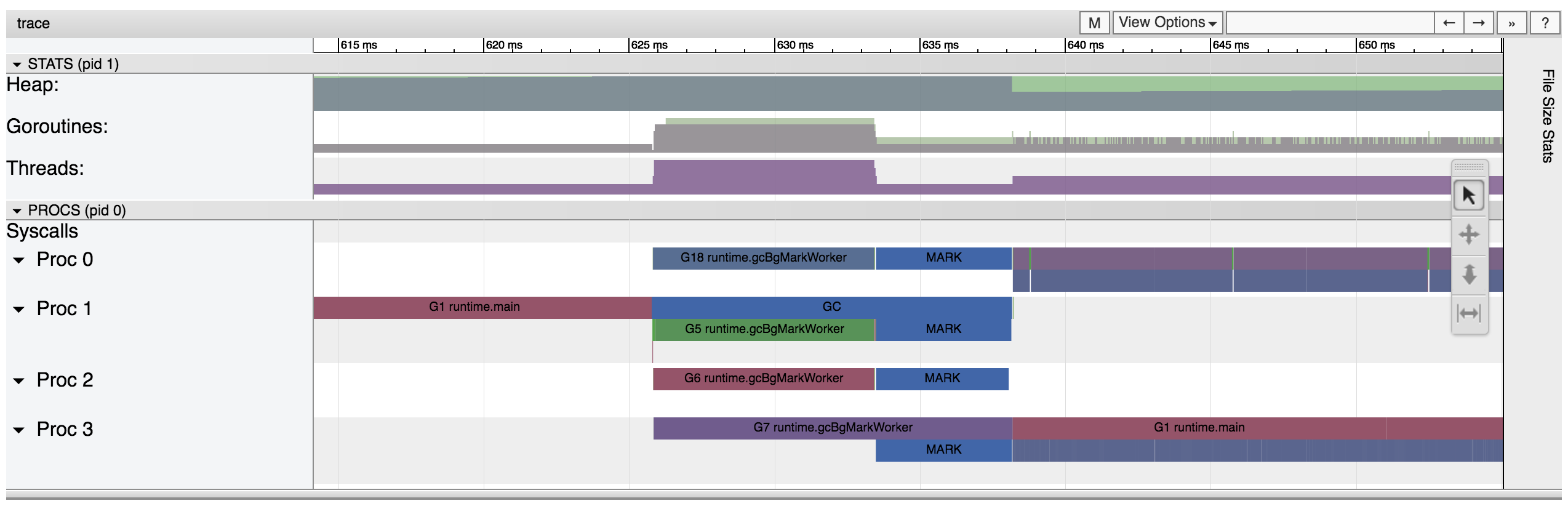## 正文

1. 不包括赋值语句(assignment statement),一个变量一旦初始化，就无法被修改(immutable)
2. 无副作用，函数除了计算结果，将不会产生任何副作用
3. 因为无副作用，所以任何表达式在任何时候都能够evaluate

``````sum [1,2,3]
-- 6
-- sum 的实现其实是
foldr (+) 0 [1,2,3]
``````

``````foldr :: Foldable t => (a -> b -> b) -> b -> t a -> b
``````

``````-- if the list is empty, the result is the initial value z; else
-- apply f to the first element and the result of folding the rest
foldr f z []     = z
foldr f z (x:xs) = f x (foldr f z xs)
``````

`foldr`函数其实做了这样的事情：`foldr`接受三个参数，第一个参数是函数`f`，第二个参数是初始值`z`,第三个参数是一个列表。如果列表为空则返回初始化值`z`，否则递归调用 `foldr`,需要说明的是函数`f`的类型是接受两个参数，返回一个值，两个参数类型都应该和`z`相同（强类型语言中）。

JavaScript

``````function foldr(f,z,list){
//为了简洁起见，把类型判断省略了
// Object.prototype,toString.call(list) === '[object Array]'
if(list === null || list.length == 0){
return z;
}
//这里的shift会改变参数的状态，会造成副作用
//return f(list.shift(),foldr(f,z,list));
//改用如下写法
return f(list,foldr(f,z,list.slice(1)));
}
``````

``````function add(a,b){
return a+b;
}
``````

``````function sum(list){
}
``````

``````let a = [1,2,3];
sum(a); // 6
``````

go

``````func foldr(f func(a,b int) int,z int,list []int)int{
if len(list) == 0{
return z
}
return f(list,foldr(f,z,list[1:]))
}
``````

go因为有数组切片，所以使用起来较为简单，但是go又是强类型的语言，所以在声明函数的时候必须要把类型声明清楚。

``````func add(a,b int) int{
return a+b;
}
``````

``````func sum(list []int) int{
}
``````

``````func main(){
a := []int{1,2,3}
sum(a) // 6
}
``````

javaScript

``````function muti(a,b){
return a*b;
}

function product(list){
return foldr(muti,1,list);
}
``````

go

``````func muti(a,b int) int{
return a*b;
}

func product(list []int) int{
return foldr(muti,1,list)
}
``````

``````foldr (*) 1 [1,2,3,4]
-- 24
-- or
myproduct xs = foldr (*) 1 xs
-- myproduct [1,2,3,4]
``````

anyTure

JavaScript

``````function or(a,b){
return a || b;
}

function anyTrue(list){
return foldr(or,false,list);
}
``````

``````let b = [true,false,true];
console.log(anyTrue(b)); // true
``````

allTure

JavaScript

``````function and(a,b){
return a && b;
}

function allTrue(list){
return foldr(and,true,list);
}
``````

``````let b = [true,false,true];
console.log(allTrue(b)); // false
``````

``````const _ = require("lodash");
_.reduce([1,2,3],function(sum,n){
return sum+n;
});
``````

`lodash`官方文档是这么定义的：

``````_.reduce alias _.foldl
_.reduceRight alias _.foldr
``````

``````foldr (-) 0 [1,2,3]
-- 输出: 2
foldl (-) 0 [1,2,3]
-- 输出: -6
``````

`foldr (-) 0 [1,2,3]`

`1-(2-(3-0)) = 2`

`foldl (-) 0 [1,2,3]`

`((0-1)-2)-3) = -6`

JavaScript

``````const _ = require("lodash");
//reduce 相当于 foldl
_.reduce([1,2,3],function(sum,n){
return sum-n;
});
// 输出 -4
``````

JavaScript

``````const _ = require("lodash");
//reduceRight 相当于 foldr
_.reduceRight([1,2,3],function(sum,n){
return sum-n;
});
// 输出 0
``````

``````f 3 (f 4 (f 5 ( f 6 z)))
-- 当 f 为 +, z = 0 上式就变为：
3 + (4 + (5 + (6 + 0)))
-- 前缀(+)形式则为：
(+)3 ((+)4 ((+)5 ((+)6 0)))
``````

``````g(g (g (g z 3) 4) 5) 6
-- 当然我们也可以类似地把 g 设为 +, z = 0， 上式就变为：
(((0 + 3) + 4) + 5) + 6
-- 改成前缀形式
(+)((+)((+)((+)0 3) 4) 5) 6
``````

reverse

``````flip' :: (a -> b -> c) -> b -> a -> c
flip' f x y= f y x
``````

Hasekll

``````foldr flip' [] [1,2,3]
``````

JavaScript

``````function flip(f, a, b){
return f(b,a);
}
//这个函数需要进行柯里化，否则无法在foldr中作为参数传入
var flip_ = _.curry(flip);

function cons(a,b){
return a.concat(b);
}

function reverse(list){
return foldr(flip_(cons),[],list);
}
``````

``````console.log(reverse([1,2,3]))
// [ 3, 2, 1 ]
``````

length

``````-- 先定义实现一个count 函数
count :: a -> b ->c
count a n = n + 1
-- 再实现一个length函数
length' = foldr (count) 0
-- 再调用
length' [1,2,3,4]
-- 4
``````

JavaScript

``````//先定义一个count函数
function count(a,n){
return n + 1;
}
//再实现length函数
function length(list){
return foldr(count,0,list);
}
//调用
console.log(length([1,2,3,4]));
// 4
``````

doubleall

``````-- 定义一个乘以2，并连接的函数
doubleandcons :: a -> [a] -> [a]
doubleandcons x y  = 2 * x : y

doubleall x = foldr doubleandcons []

-- 调用
doubleall [1,2,3]
-- 输出
-- [2,4,6]

``````

JavaScript

``````function doubleandcons(a,list){
return [a * 2].concat(list)
}

function doubleall(list){
return foldr(doubleandcons,[],list)
}

//调用
console.log(doubleall([1,2,3]));
// [2,4,6]
``````

go

go 的尴尬之处在于，需要非常明确的函数定义，所以我们要重新写一个`foldr`函数，来接受第二个参数为列表的`f`

``````func foldr2(f func(a int,b []int) []int,z []int,list []int)[]int{
if len(list) == 0{
return z
}
return f(list,foldr2(f,z,list[1:]))
}
``````

``````func doubleandcons(n int,list []int) []int{
return append([]int{n * 2},list...)
}

func doubleall(list []int) []int{
return foldr2(doubleandcons,make([]int,0),list)
}
// doubleall([]int{1,2,3,4})
//[2 4 6 8]
``````

go这门强类型编译语言虽然支持一定的函数式编程，但是使用起来还是有一定局限性的，起码代码复用上还是不如js的。

``````fandcons f el [a]= (f el) : [a]
double el = el * 2
-- 只传入部分参数，柯里化
doubleandcons = fandcons double
``````map

``````fandcons :: (a->b) ->a -> [b] -> [b]
fandcons f x y= (f x):y

map' :: (a->b) -> [a] -> [b]
map' f x = foldr (fandcons f) [] x

-- 调用
map' (\x -> 2 * x)  [1,2,3]
-- 输出 [2,4,6]
``````

JavaScript

``````function fandcons(f, el, list){
return [f(el)].concat(list);
}
//需要柯里化
var fandcons_ = _.curry(fandcons);

function map(f, list){
return foldr(fandcons_(f),[],list);
}
//调用
console.log(map(function(x){return 2*x},[1,2,3,4]));
// 输出[ 2, 4, 6, 8 ]
``````

summatrix

``````summatrix :: Num a => [[a]] -> a
summatrix x = sum (map sum x)

-- 调用
summatrix [[1,2,3],[4,5,6]]
-- 21
``````

JavaScript

``````function sum(list){